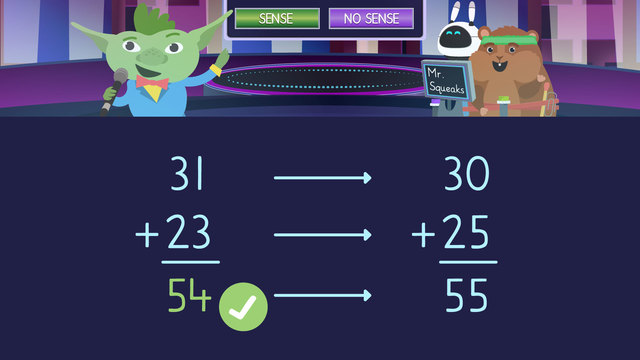# Estimation in Word ProblemsRating

Ø 5.0 / 2 ratings
The authorsTeam Digital
Estimation in Word Problems
CCSS.MATH.CONTENT.4.NBT.A.3

## Basics on the topicEstimation in Word Problems

***Estimation in Word Problems

In word problems, checking our work for reasonableness is a useful skill. We can use estimation strategies such as rounding or compatible numbers with mental computation. First, read and then reread the problem to identify the numbers used for computation. Then, use rounding or compatible numbers. Next, use mental math to find an estimate. Finally, compare the estimation to the original answer to determine reasonableness. If the numbers are close, your math is most likely correct. If the numbers are far apart, your math most likely has a mistake and needs to be corrected.

## Estimation in Word Problems exercise

Would you like to apply the knowledge you’ve learned? You can review and practice it with the tasks for the video Estimation in Word Problems .
• ### Outline the steps for checking a word problem.

Hints

In any process, the first step is always to look at, observe, or read the information.

When solving a math problem, calculating the answer always comes before checking the answer.

Solution

When using estimation in word problems:

• First read, then re-read the problem, to identify the numbers used for computation.
• Second, use rounding or compatible numbers to simplify the problem.
• Third use mental math to find an estimate.
• Last compare the estimate to the original answer to determine if it is reasonable, or not.

• ### Is the answer reasonable, or not?

Hints

When rounding up or making compatible numbers always estimate to the closest 5 or 10.
Examples:
17 becomes 20.
63 becomes 65.

Compare your estimation to the original answer to determine if it is reasonable or not.

If there is a large difference, more than 20 or so, then it is not reasonable.

Solution
• Zuri collected 6 boxes with 24 cans in each.
24 can be rounded up to 25.
6 x 25 = 150

• Freddie collected 4 boxes with 37 cans in each.
37 can be rounded up to 40.
4 x 40 = 160

• Freddie has about 160 cans.
160 - 150 = 10.
Freddie has about 10 cans more than Zuri.

• Freddie's claim that he collected 292 more cans than Zuri is not reasonable.
• ### Determine how to solve the word problem.

Hints

When rounding up or making compatible numbers always estimate to the closest 5 or 10.
Examples:
17 becomes 20.
63 becomes 65.

The word each in a word problem signals that multiplication should be used.
Each of Bill's 23 guests receives 2 small toys.
23 x 2 = the number of toys Bill and Marty need to buy.

There is only one correct choice.

Solution
• Bill invited 23 guests, so round 23 up to 25 to make it friendlier.
• Bill wants to give 2 small toys to each guest, so multiply 25 by 2.
• 2 x 25 = 50.
• ### Solve the word problem using estimation.

Hints

When rounding or making compatible numbers always estimate to the closest 5 or 10.
Examples:
17 becomes 20.
63 becomes 65

Compare your estimation to the original answer to determine if it is reasonable or not.

If there is a large difference, more than 20 or so, then it is not reasonable.

Solution

1) $5.85 can be rounded down to$5.00.

$14.90 can be rounded up to$15.00.

2) $5.00 x 11 =$55.00.

$15.00 x 10 =$150.00.

3) $55.00 +$150.00 = $205.00. Marie's guess of$300.00 for flowers does not make sense.

• ### Use mental math to make compatible numbers.

Hints

When rounding up or making compatible numbers always estimate to the closest 5 or 10.
Examples:
17 becomes 20.
63 becomes 65.

When rounding up or making compatible numbers with money, always eliminate the cents and work only with full dollars.
Examples:
$7.83 becomes$10.00.

$22.99 becomes$25.00.

Solution
• $67.99 is rounded up to$70.00.
• $53.99 is rounded up to$55.00.
• $38.99 is rounded up to$40.00.
• $42.99 is rounded up to$45.00.
• ### Use estimation to solve the word problem.

Hints

When rounding up or making compatible numbers always estimate to the closest 5 or 10.
Examples:
17 becomes 20.
63 becomes 65

To find how many pens Drew needs in total, multiply 10 (each person gets 10 pens) by the number of people who need them. Use the rounded number to make the problem friendlier!

To find how many packs of pens Drew needs, take the total number of pens needed and divide by 5. (There are 5 pens in each pack).

For the final question, write either 'reasonable' or 'not reasonable'.

Solution
• 4 people can be rounded up to 5 to make the number friendlier.
• 10 pens x 5 people = 50.
• 50 pens ÷ 5 = 10.
• Drew's guess that he needs 10 packs of pens is reasonable.